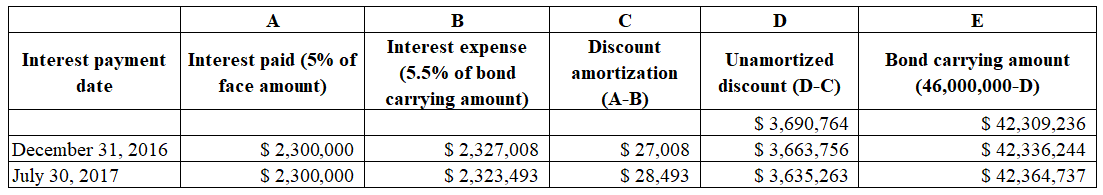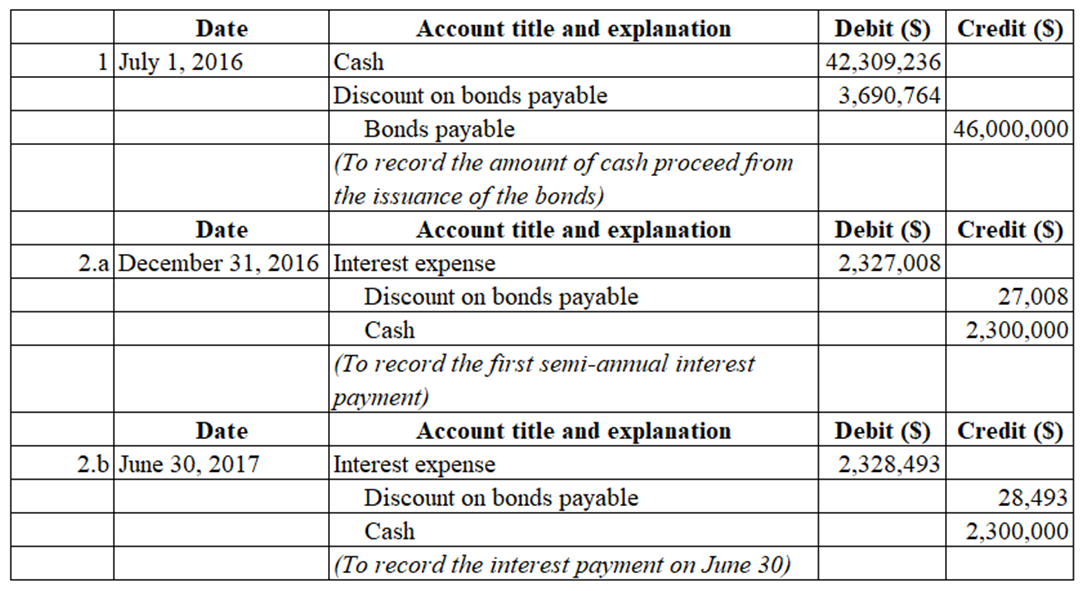# On July 1, Year 1, Livingston Corporation, a wholesaler of manufacturing equipment, issued \$46,000,000 of 20-year, 10% bonds at a market (effective) interest rate of 11%, receiving cash of \$42,309,236. Interest on the bonds is payable semiannually on December 31 and June 30. The fiscal year of the company is the calendar year.Required:For all journal entries with a compound transaction, if an amount box does not require an entry, leave it blank.1.  Journalize the entry to record the amount of cash proceeds from the issuance of the bonds on July 1, Year 1. For a compound transaction, if an amount box does not require an entry, leave it blank.2.  Journalize the entries to record the following:a.   The first semiannual interest payment on December 31, Year 1, and the amortization of the bond discount, using the straight-line method. (For a compound transaction, if an amount box does not require an entry, leave it blank. Round to the nearest dollar.)b.   The interest payment on June 30, Year 2, and the amortization of the bond discount, using the straight-line method. (For a compound transaction, if an amount box does not require an entry, leave it blank. Round to the nearest dollar.)3.  Determine the total interest expense for Year 1. Round to the nearest dollar.4.  Will the bond proceeds always be less than the face amount of the bonds when the contract rate is less than the market rate of interest?5.  Compute the price of \$42,309,236 received for the bonds by using the Table 1, Table 2, Table 3 and Table 4. (Round to the nearest dollar.) Your total may vary slightly from the price given due to rounding differences.Present value of the face amount\$Present value of the semiannual interest payments Price received for the bonds

Question
36 views

On July 1, Year 1, Livingston Corporation, a wholesaler of manufacturing equipment, issued \$46,000,000 of 20-year, 10% bonds at a market (effective) interest rate of 11%, receiving cash of \$42,309,236. Interest on the bonds is payable semiannually on December 31 and June 30. The fiscal year of the company is the calendar year.

Required:

For all journal entries with a compound transaction, if an amount box does not require an entry, leave it blank.

1.  Journalize the entry to record the amount of cash proceeds from the issuance of the bonds on July 1, Year 1. For a compound transaction, if an amount box does not require an entry, leave it blank.

2.  Journalize the entries to record the following:

a.   The first semiannual interest payment on December 31, Year 1, and the amortization of the bond discount, using the straight-line method. (For a compound transaction, if an amount box does not require an entry, leave it blank. Round to the nearest dollar.)

b.   The interest payment on June 30, Year 2, and the amortization of the bond discount, using the straight-line method. (For a compound transaction, if an amount box does not require an entry, leave it blank. Round to the nearest dollar.)

3.  Determine the total interest expense for Year 1. Round to the nearest dollar.

4.  Will the bond proceeds always be less than the face amount of the bonds when the contract rate is less than the market rate of interest?

5.  Compute the price of \$42,309,236 received for the bonds by using the Table 1, Table 2, Table 3 and Table 4. (Round to the nearest dollar.) Your total may vary slightly from the price given due to rounding differences.

 Present value of the face amount \$ Present value of the semiannual interest payments Price received for the bonds

check_circle

Step 1

Hey, since there are multiple sub-parts are posted, we will answer first three sub-parts. If you want any specific sub-part to be answered then please submit that sub-part only or specify the sub-part number in your message.

Step 2

Step 1: Calculation of amortization of discount on bonds payable:Step 3

Step 2: Record the journal entries for 1, 2a, and 2b....

### Want to see the full answer?

See Solution

#### Want to see this answer and more?

Solutions are written by subject experts who are available 24/7. Questions are typically answered within 1 hour.*

See Solution
*Response times may vary by subject and question.
Tagged in